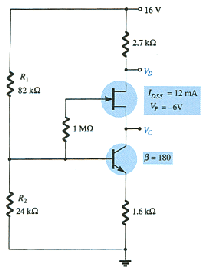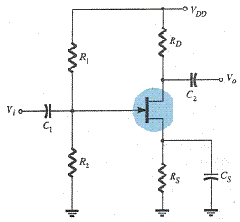# Practice Quiz in DC Biasing – FET Part 6

(Last Updated On: February 3, 2020)

This is the Online Practice Quiz in DC Biasing – FETs Part 6 from the book, Electronic Devices and Circuit Theory 10th Edition by Robert L. Boylestad. If you are looking for a reviewer in Electronics Engineering this will definitely help. I can assure you that this will be a great help in reviewing the book in preparation for your Board Exam. Make sure to familiarize each and every questions to increase the chance of passing the ECE Board Exam.

### Continue Part VI of the Online Practice Quiz

#### Quiz in DC Biasing – FETs

Question 51. In a fixed-bias configuration, the voltage level of VGS is equal to _____.

A. VS

B. VG

C. VGS(off)

D. VP

Question 52. The dc load line is drawn using the equation obtained by applying Kirchhoff's voltage law (KVL) at _____ side loop(s) of the circuit.

A. the output

B. the input

C. both the input and output

D. None of these

Question 53. For R2 smaller than _____ kΩ the voltage VD is equal to VDD = 16 V.A. 3.75

B. 5

C. 12.0

D. 24

Question 54. The slope of the dc load line in a self-bias configuration is controlled by _____.

A. VDD

B. RD

C. RG

D. RS

Question 55. The controlled variable on the output side of an FET transistor is a _____ level.

A. current

B. voltage

C. resistor

D. None of these

Question 56. In a feedback-bias configuration, the slope of the dc load line is controlled by _____.

A. RG

B. RD

C. VDG

D. None of these

Question 57. In p-channel FETs, the level of VGS is _____ while the level of VDS is _____.

A. negative, negative

B. positive, positive

C. negative, positive

D. positive, negative

Question 58. The level of VDS is typically between _____ % and _____ % of VDD.

A. 0, 100

B. 10, 90

C. 25, 75

D. None of these

Question 59. In a universal JFET bias curve, the vertical scale labeled m is used to find the solution to the _____ configuration.

A. fixed-bias

B. self-bias

C. voltage-divider

D. None of these

Question 60. The slope of the dc load line in a voltage-divider is controlled by _____.A. R1

B. R2

C. RS

D. All of these

### More Practice Quiz in DC Biasing – FETs

Practice Quiz Part 1

Practice Quiz Part 2

Practice Quiz Part 3

Practice Quiz Part 4

Practice Quiz Part 5

Practice Quiz Part 6

### See: Complete List of Practice Quizzes

Note: After taking this particular quiz, you can proceed to check all the topics.

Please do Subscribe on YouTube!

P inoyBIX educates thousands of reviewers and students a day in preparation for their board examinations. Also provides professionals with materials for their lectures and practice exams. Help me go forward with the same spirit.

“Will you subscribe today via YOUTUBE?”

Subscribe